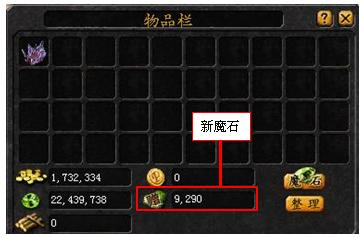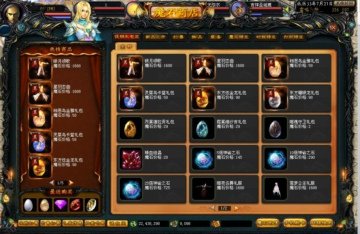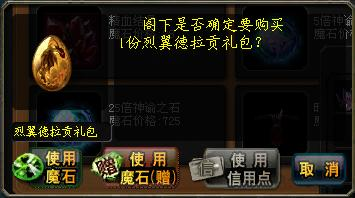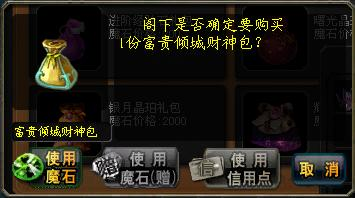# 新魔石使用指南

新魔石是指不可交易的特殊魔石，并且附带有“赠”属性，新魔石所购买的东西都是带有 “赠”属性的物品。
新魔石会区别一般魔石存放在您的背包中。新魔石是不能直接充值获取的，玩家可以通过参加活动或是系统赠予等方式获得。

新魔石只可在商城内消费，可以购买商城内绝大部分的物品，但是对个别物品有购买限制。限制购买物品如下：

 限制物品 类别 魔石 新魔石 限制物品 类别 魔石 新魔石 元素紊乱 技能书 √ X 神圣月光宝盒 道具 √ X 暗影诅咒 √ X 回初魔剂 √ X 幻影移行 √ X 结界水晶 √ X 幻影神术 √ X 死灵契约书 √ X 真视打击 √ X 孕灵 √ X 迷心术 √ X 圣佑草 √ X 炼血化灵 √ X 铜质启蒙学币 √ X 血袭 √ X 银质启蒙学币 √ X 瞬影击 √ X 金质启蒙学币 √ X 血翼天袭 √ X 儿童肥牛排 √ X 血舞影煞 √ X 启灵雪参 √ X 血域风暴 √ X 承爱心火 √ X 暗沙邪龙 √ X 四千点图鉴卡 √ X 冥国圣女 √ X 六阶兽魂原石 √ X 亡念巫灵 √ X 战场复活药 √ X 黑龙舞 √ X 洗孕灵泉 √ X 永夜极爆 √ X 神祈之泪 √ X 降灵咒雨 √ X 神谕之石 √ X 召唤巫环 √ X 神炼之符 √ X 焰魂枪·断空 √ X 创世晶石 √ X 焰魂枪·幻灭 √ X 曙光战魂 √ X 骑士团守护 √ X 帝龙之泪 √ X 碎星钻 √ X 圣耀符文 √ X 炽链陨灭 √ X 12星XO神兽礼包 礼包 √ X 灭世审判 √ X 6星O型神兽礼包 √ X 生命祈祷 √ X 圣诞珍宝盒 √ X 座骑禁锢 √ X 12星XO礼包 √ X 虚弱诅咒 √ X 6星O礼包 √ X 幻影诅咒·暗 √ X 炫光黄金马礼包 √ X 重力束缚 √ X 星痕水晶马礼包 √ X 神罚 √ X 豪华富贵包 √ X 火球术 √ X 富贵倾城财神包 √ X 潜行 √ X 新手经验石礼包 √ X 霹雳火 √ X 成长经验石礼包 √ X 幽冥鬼火 √ X 冒险经验石礼包 √ X 光辉光环 √ X 进阶经验石礼包 √ X 灵魂净化 √ X 曙光晶珀礼包 √ X 诱捕陷阱 √ X 晨星晶珀礼包 √ X 风斩 √ X 银月晶珀礼包 √ X 斩魂 √ X 耀阳晶珀礼包 √ X 四连斩 √ X 水晶花语礼包 √ X 星魔剑 √ X 军团战旗礼包 √ X 六连斩 √ X 心语真爱焰火包 √ X 重击 幻兽技能 √ X 水晶幻恋焰火包 √ X 剑气斩 √ X 倾城之恋焰火包 √ X 旋风斩 √ X 绝恋倾城焰火包 √ X 引诱 √ X 梦幻·圣诞衣 外套及发型 √ X 爆炎斩 √ X 罗曼幻恋礼服 √ X 霹雳弹 √ X 活力盛夏 √ X 爆雷术 √ X 清凉盛夏 √ X 电击术 √ X 南瓜小礼帽 √ X 雷霆万钧 √ X 南瓜怪盗礼包 √ X 风珠 √ X 南瓜公主礼包 √ X 暗影突袭 √ X 火雉蓝翎面罩 √ X 死亡旋舞 √ X 火雉红冠面罩 √ X 破空斩 √ X 火雉王子礼包 √ X 光芒之刃 √ X 火雉女王礼包 √ X 死神裁决 √ X 梦幻·圣诞帽 √ X 破灵咒 √ X 圣诞驯鹿冠 √ X 噬魂咒 √ X

商城使用新魔石消费状态如下：四、新魔石使用限制及注意事项：

１．新魔石是不可以交易流通的，所购买的物品也是不可交易的“赠”属性物品哦。
（商业伙伴也无法交易）

２．15星以下O型和XO型宠做为主幻兽与不可交易的副幻兽幻化时，主幻兽将会转变为不可交易属性。

３．“赠品”物品的限制如下：

 赠品中级宝石无法合成高级宝石 赠品幻魔晶石无法合成幻魔之心 赠品灵魂晶石无法合成灵魂王 赠品魔魂晶石无法合成魔魂之心 赠品宝钻无法与非赠品装备进行锻造 赠品装备无法兑换圣兽魔晶 赠品物品丢弃后消失 赠品灵魂晶石无法为武器开洞 赠品法宝无法与非赠品法宝进行锻造

４．新魔石使用过程不可参与以下行为：

 限制行为 魔石 新魔石 摊位购买物品 √ X 交易流通 √ X 求购，拍卖 √ X 军团捐献 √ X 摊位魔石 √ X 换蛋，批量孵化 √ X 摊位并购抵扣 √ X 城堡升级 √ X